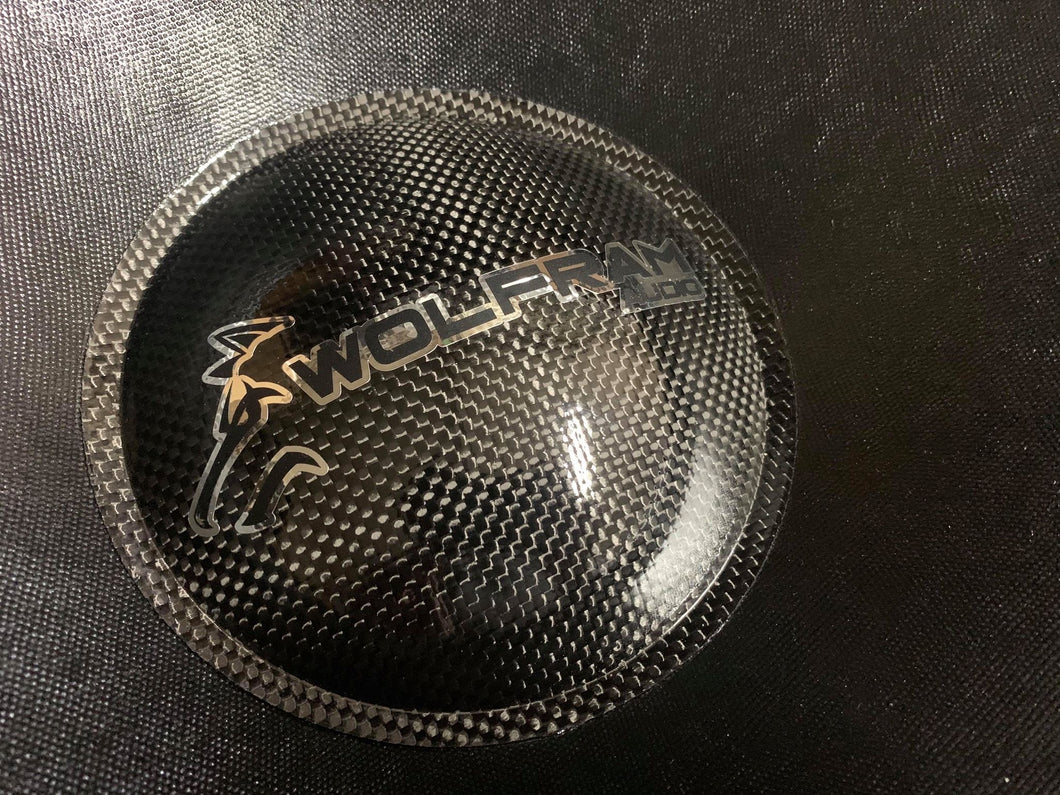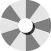# Pt-15 | 15" SPL Subwoofer

Regular price \$699.95 Sale

MADE IN USA

Wolfram Audio is proud to introduce the most powerful subwoofer in our lineup : the Platinum series. With such a high continuous power handling, this subwoofer was engineered to be an absolute demo beast. The Platinum series features a 450 oz motor and 4 inch flatwound aluminum PE coil.Specifications:

• Wolfram Audio Pt-15 - 15" SPL Subwoofer
• Dual 1 or 2 ohm
• RMS Power: 4,000 Watts
• Peak Power: 8,000 Watts
• Voice Coil: 4" - Flatwound Aluminum PE Coil
• High Roll Foam Surround
• 4 Gauge Push Terminals
• XMAX: 33mm (One Way)

### Thiele/Small Parameters

Dual 1 Ohm
• Re = 1.2443 ohms
• Fs = 28.2942 Hz
• Qes = 0.4166
• Qms = 6.3785
• Qts = 0.3911
• Le = 1.5070 mH (at 1 kHz)
• Vas = 73.8364 L ( 2.6075 ft^3)
• Sd = 79173.0434 mm^2(122.7185 in^2)
• BL = 14.2343 N/A
• Mms = 381.5865 g
• Cms = 82.9185 uM/N
• Sens = 87.7838 dB @1W/1m

Dual 2 Ohm

• Re = 2.9266 ohms
• Fs = 28.5163 Hz
• Qes = 0.4239
• Qms = 6.2632
• Qts = 0.3970
• Le = 3.1894 mH (at 1 kHz)
• Sd = 79173.0434 mm^2(122.7185 in^2)
• Vas = 73.8599 L ( 2.6083 ft^3)
• BL = 21.5543 N/A
• Mms = 375.5471 g
• Cms = 82.9449 uM/N
• Sensitivity= 87.8121 dB @1W/1m
Spin to win